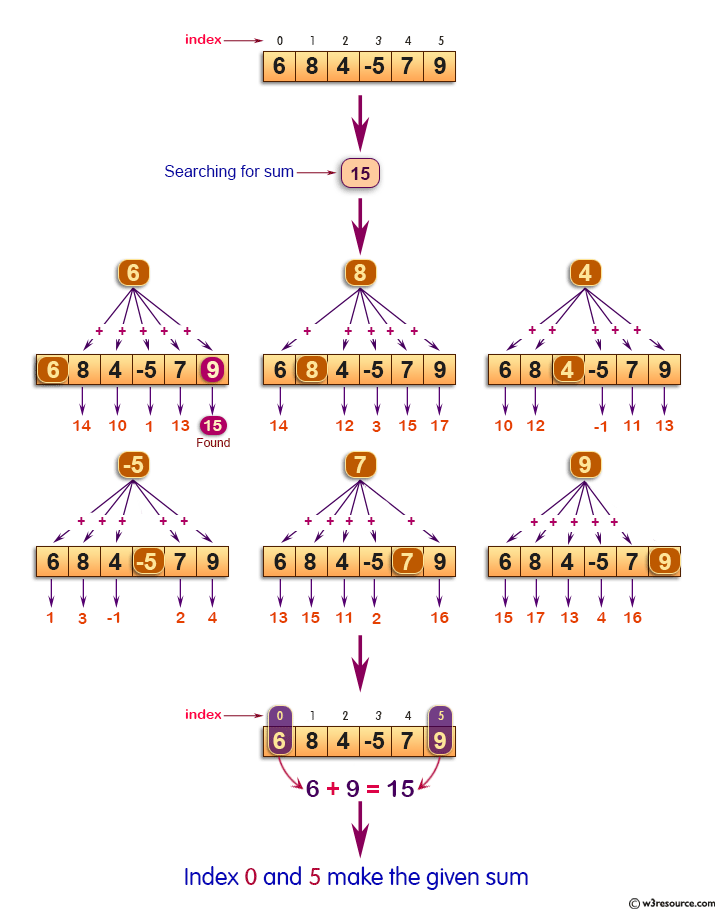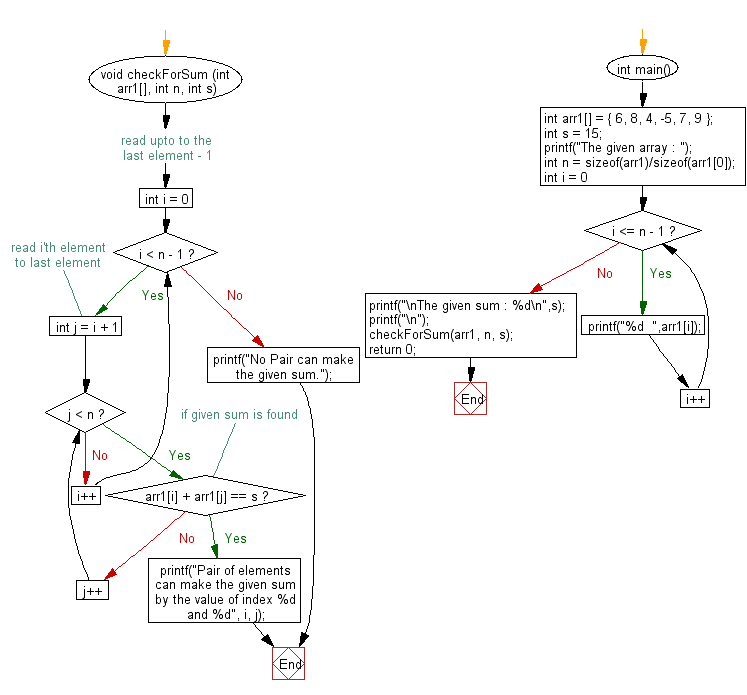﻿ C exercises: Find a pair with given sum in the array - w3resource# C Exercises: Find a pair with given sum in the array

## C Array: Exercise-32 with Solution

Write a program in C to find a pair with given sum in the array.

Pictorial Presentation:Sample Solution:

C Code:

``````#include <stdio.h>
void checkForSum (int arr1[], int n, int s)
{
// read upto to the last element - 1
for (int i = 0; i < n - 1; i++)
{
// read i'th element to last element
for (int j = i + 1; j < n; j++)
{
// if given sum is found
if (arr1[i] + arr1[j] == s)
{
printf("Pair of elements can make the given sum by the value of index %d and %d", i, j);
return;
}
}
}
printf("No Pair can make the given sum.");
}

int main()
{
int arr1[] = { 6, 8, 4, -5, 7, 9 };
int s = 15;
printf("The given array : ");
int n = sizeof(arr1)/sizeof(arr1);
for (int i = 0; i <= n - 1; i++)
{
printf("%d  ",arr1[i]);
}
printf("\nThe given sum : %d\n",s);
printf("\n");
checkForSum(arr1, n, s);
return 0;
}
```
```

Sample Output:

```The given array : 6  8  4  -5  7  9
The given sum : 15

Pair of elements can make the given sum by the value of index 0 and 5
```

Flowchart :C Programming Code Editor:

Improve this sample solution and post your code through Disqus.

What is the difficulty level of this exercise?

﻿